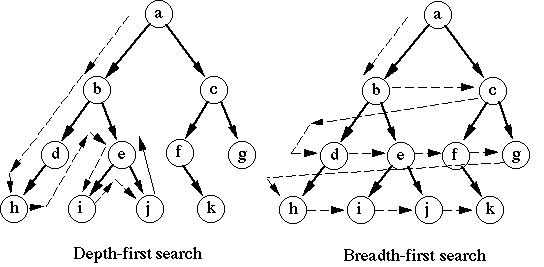# Tree Traversal

## BFS vs. DFS

When you need to search or traverse a graph or tree-like structure, there are two common algorithms: breadth-first search and depth-first search.Which algorithm to use really depends on the structure of the tree and the location of the items you need to find. Here are some quick guidelines (not steadfast rules) for determining which algorithm to use:

• The target is not far from the root
• You are looking for the shortest path from the root
• You have a lot of memory to work with

Depth-first:

• The target is deep in the tree
• You don't care for the shortest path from the root
• The tree is very wide and you have limited memory

## Sample Code

``````var Tree = function(value) {
this.value = value;
this.children = [];
};

// Assume we have helper functions to add and remove children
Tree.prototype.addChild = function(child) {/* Code to add child */};
Tree.prototype.removeChild = function(child) {/* Code to remove child */};

Tree.prototype.BFS = function(filter) {
var queue = [this]; // The queue of nodes to check, starting with the current node

while (queue.length) {
var node = queue.shift(); // Remove and examine first element in queue
if (filter(node.value)) return node; // Found target node
queue = queue.concat(node.children); // Node is not target, add its children to the queue
}

return null; // No result found
};

// Depth-first search
Tree.prototype.DFS = function(filter) {
if (filter(this.value)) return this; // Found target node

for (var i = 0; i < this.children.length; i++) {
var search = this.children[i].DFS(filter); // Recurse down the tree
if (search) return search;
}

return null; // No result found
};
``````

Now let's say our tree contains nodes that hold a number, and we want to find the first node with a value divisible by 5. Here is how we can set up that tree and use BFS and DFS to find the target node:

``````var root = new Tree(1);
var branch1 = root.addChild(2);
var branch2 = root.addChild(3);
var branch3 = branch1.addChild(4);
var branch4 = branch1.addChild(5);
var leaf1 = branch2.addChild(6);
var leaf2 = branch2.addChild(7);
var leaf3 = branch3.addChild(8);
var leaf4 = branch3.addChild(9);
var leaf5 = branch4.addChild(10);

var filter = function(value) {
return value % 5 === 0;
};

var node = root.BFS(filter); // Returns the node with value 5
var node = root.DFS(filter); // Returns the node with value 5
``````

When trying to find the first node divisible by 5 using BFS, this is the order of which the nodes are examined: `1 -> 2 -> 3 -> 4 -> 5`. And when using DFS: `1 -> 2 -> 4 -> 8 -> 9 -> 5`.